Next: Spherical Coordinates Up: Non-Cartesian Coordinates Previous: Orthogonal Curvilinear Coordinates

Cylindrical Coordinates

In the cylindrical coordinate system,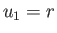,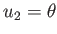, and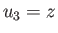, where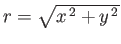,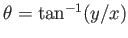, and,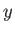,are standard Cartesian coordinates. Thus,is the perpendicular distance from the-axis, and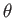the angle subtended between the projection of the radius vector (i.e., the vector connecting the origin to a general point in space) onto the-plane and the-axis. (See Figure C.1.)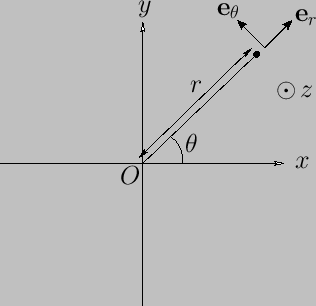A general vector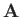is written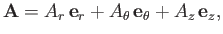(C.29)

where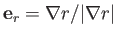,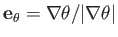, and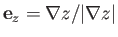. Of course, the unit basis vectors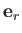,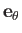, and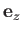are mutually orthogonal, so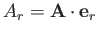, et cetera.

As is easily demonstrated, an element of length (squared) in the cylindrical coordinate system takes the form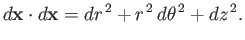(C.30)

Hence, comparison with Equation (C.6) reveals that the scale factors for this system are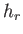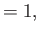(C.31)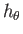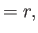(C.32)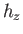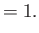(C.33)

Thus, surface elements normal to,, andare written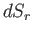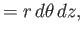(C.34)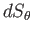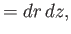(C.35)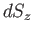(C.36)

respectively, whereas a volume element takes the form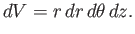(C.37)

According to Equations (C.13), (C.15), and (C.18), gradient, divergence, and curl in the cylindrical coordinate system are written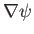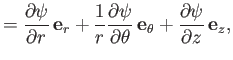(C.38)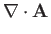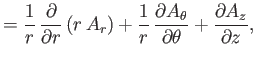(C.39)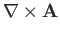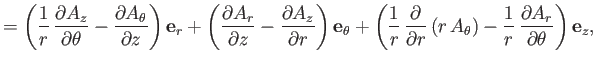(C.40)

respectively. Here,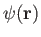is a general scalar field, and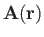a general vector field.

According to Equation (C.19), when expressed in cylindrical coordinates, the Laplacian of a scalar field becomes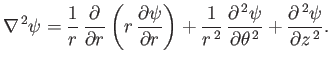(C.41)

Moreover, from Equation (C.23), the components of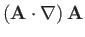in the cylindrical coordinate system are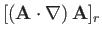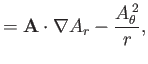(C.42)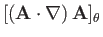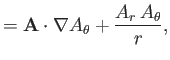(C.43)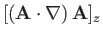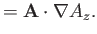(C.44)

Let us define the symmetric gradient tensor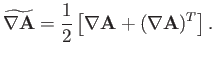(C.45)

Here, the superscript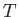denotes a transpose. Thus, if the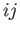element of some second-order tensor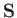is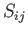then the corresponding element of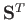is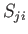. According to Equation (C.26), the components of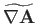in the cylindrical coordinate system are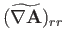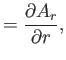(C.46)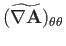(C.47)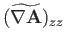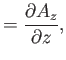(C.48)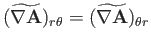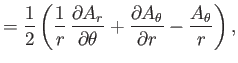(C.49)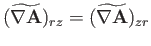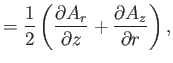(C.50)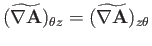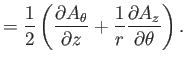(C.51)

Finally, from Equation (C.28), the components of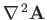in the cylindrical coordinate system are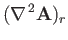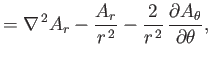(C.52)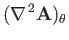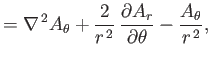(C.53)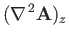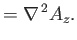(C.54)Next: Spherical Coordinates Up: Non-Cartesian Coordinates Previous: Orthogonal Curvilinear Coordinates
Richard Fitzpatrick 2016-01-22